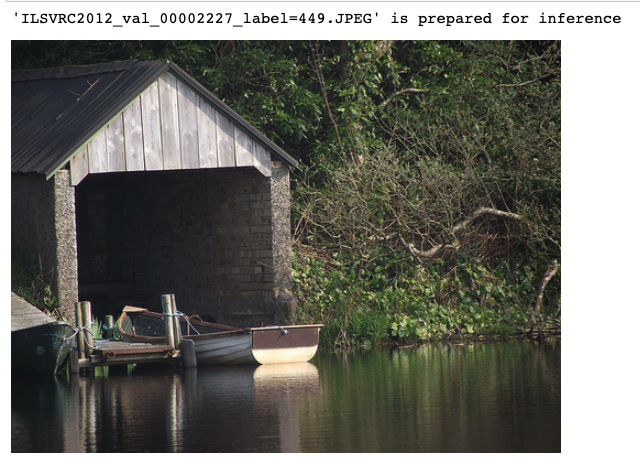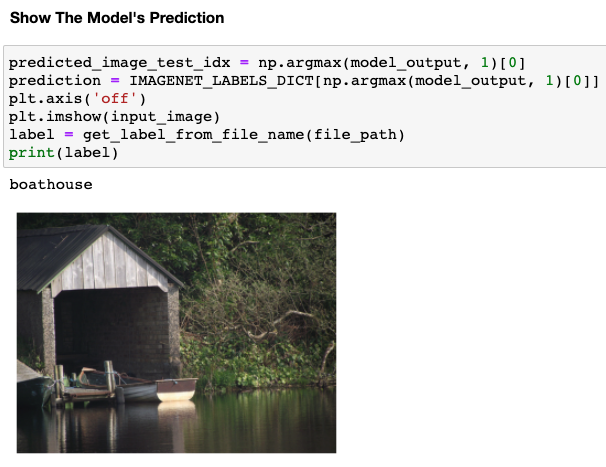# Running Inference with INFERY

Once we loaded the model (see how), we are ready for inference.

In the example below, inputs represents the input tensor to be processed by the model. In this example, it is an automatically generated random tensor, which should be replaced by the real tensor to be processed by the model.predict command.

``````# model = infery.load(weights_path='/tmp/model.onnx', framework_type='onnx')

# Define the input tensor. For demonstration, we create a tensor with random values.
inputs = np.random.random((1, 3, 224, 224)).astype('float32')

# Run inference on the model with inputs.
model.predict(inputs)
``````

### 👍Keyword Arguments

Infery supports chaining **kwargs to benchmark() and predict().
When extra arguments are passed to the function, so they are passed to your model's framework, as-is.

The output of the model is a numpy.ndarray, with shape (1, 1000), because the model was trained on the Imagenet dataset, that is composed of 1000 labels –

``````[array([[-2.16313553e+00, -7.49338865e-01, -4.13975000e-01,
-5.33734620e-01, -6.56776190e-01, -1.02638006e+00,
-1.13409054e+00, -9.78322923e-01, -1.32272959e+00,
-1.02403033e+00,  6.21275842e-01,  1.09605193e+00,
...
2.25341439e+00]], dtype=float32)]
``````

Infery will always return a list of numpy arrays as a result.

## Running Inference on an Image

``````# Load a real image using PIL, Pillow, Torch or any other utility.
input_image , file_path = load_random_example_image(image_dir='./example_images/', img_extension='JPEG')
display(input_image)
````````````model_np_input = preprocess_image(input_image) # PREPROCESSING FOR IMAGENET FORMAT
model_outputs = model.predict(model_np_input) # RUNNING INFERENCE ON THE ARRAY
model_output = model_outputs # INFERY RETURNS A LIST OF OUTPUTS, WE CHOOSE THE RIGHT ONE FOR IMAGENET.
``````